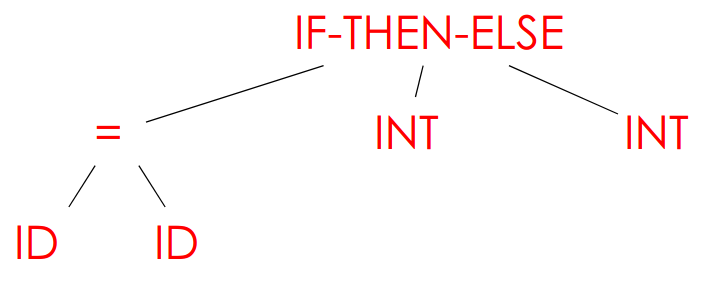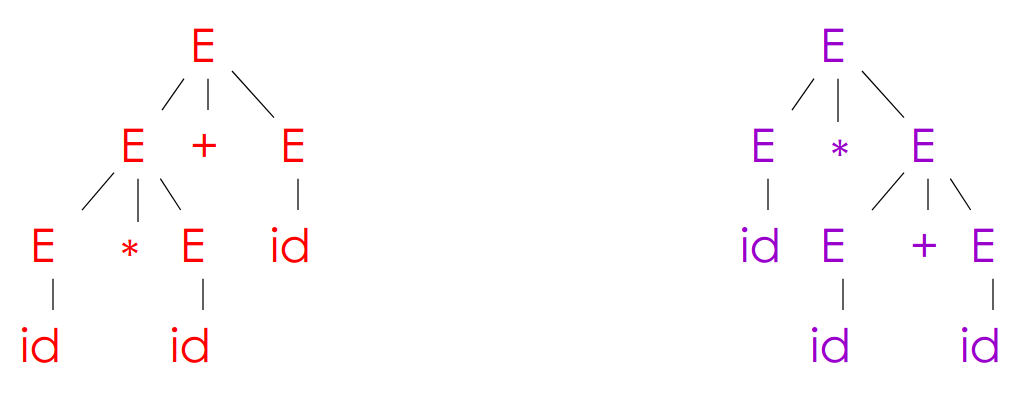Parsing

# Parsing #

## Parser functionality #

• Input: sequence of tokens from lexer
• Output: parse tree of the program (in practice, Abstract Syntax Tree)
• Parser distinguishes between valid and invalid strings of tokens
• Requires a language for describing valid strings of tokens
• Requires a method for distinguishing between valid and invalid strings of tokens

e.g.

• COOL syntax: `if x = y then 1 else 2 fi`
• Parser input (Lexer output): `IF ID = ID THEN INT ELSE INT FI`
• Parser output:## Context-Free Grammars #

• Many languages (i.e., balanced parenthesis) are nonregular yet we want to be able to capture them
• A finite automaton that runs long enough must repeat states, but finite automata can’t remember the number of times it visited a state

A CFG is a natural notation for recursive structure found in programming language constructs

• e.g. an `EXPR` can be
• `if EXPR then EXPR else EXPR fi`
• `while EXPR loop EXPR pool`
• A CFG consists of
• A set of terminals `T`
• A set of non-terminals `N`
• A start symbol `S` (a non-terminal)
• A set of productions X -> Y1Y2…Yn, where
• X in N
• Yi in T ∪ N ∪ {ε}
• COOL example:
``````EXPR -> if EXPR then EXPR else EXPR fi
| while EXPR then EXPR pool
| id
``````
• Implementation: Bison

### CFG languages #

• Read productions as rules: X -> Y1Y2…Yn means X can be replaced by Y1Y2…Yn
• Key idea:
1. Begin with a string consisting of the start symbol `S`
2. Replace non-terminals \$X\$ in the string by the right-hand side of some production X -> Y1Y2…Yn
3. Repeat (2) until there are no non-terminals left in the string
• Formally: Let \$G\$ be a CFG with start symbol \$S\$. Then the language of \$G\$ is {a1…an | S ->* a1…an and every ai is a terminal}
• Terminals are so-called because there are no rules for replacing them: once generated, they are permanent
• Terminals should be tokens of the language
• Example: strings of balanced parantheses
• S -> (S), S -> ε
• Example: arithmetic expressions
• E -> E+E | E*E | (E) | id

## Derivations and parse trees #

• A derivation is a sequence of productions leading to a string of only terminals: s -> … d -> …
• A derivation can be drawn as a tree
• Start symbol is a tree’s root
• For a production X -> Y1Y2…Yn add children X -> Y1Y2…Yn to node X
• Example:• Notes:
• A parse tree has terminals at the leaves, non-terminals at the inner nodes
• An in-order traversal of the leaves is the original inputs
• The parse tree shows the association of operations, the input string does not
• Can do derivations in left-most order (replace left-most non-terminal at each step) or right-most order; these yield equivalent parse trees

### Ambiguity #

• A CFG is ambiguous if it has more than one parse tree for some string
• Leaves meaning of some programs ill-defined
• Example:
• Grammar E -> E+E | E*E | (E) | id
• String id * id + id
• Yields following parse trees:• To deal with ambiguity, most direct method is to rewrite grammar unambiguously
• E -> E’ + E | E'
• E -> id * E’ | id | (E) * E’ | (E)
• Enforces precedence of multiplication over addition
• Tools now can allow precedence and associativity declarations to allow one to write natural ambiguous grammar and then disambiguate later